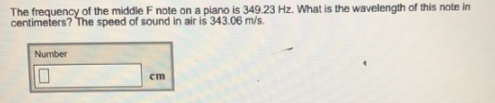# Problem: The frequency of the middle F note on a piano is 349.23 Hz. What is the wavelength of this note in centimeters? The speed of sound in air is 343.06 m/s.

###### FREE Expert Solution
99% (399 ratings)###### Problem Details

The frequency of the middle F note on a piano is 349.23 Hz. What is the wavelength of this note in centimeters? The speed of sound in air is 343.06 m/s.